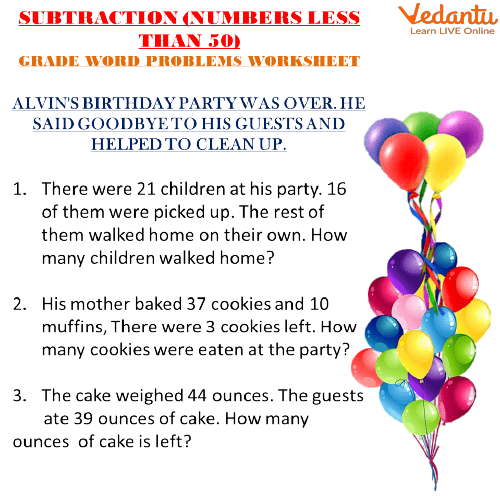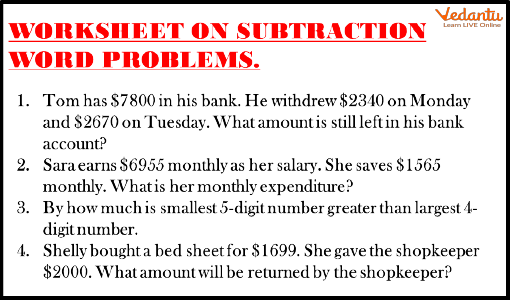Courses
Courses for Kids
Free study material
Offline Centres
More

# Subtraction Word ProblemsLast updated date: 27th Nov 2023
Total views: 143.1k
Views today: 4.43k## Introduction and Basic Rules of Subtraction

Subtraction is used to calculate the remaining number from the two given numbers. There are certain basic rules to the concept of subtraction word problems.

We have to find the larger number out of the two given numbers. Then we have to find out the number which has to be subtracted from the larger number. We always start by subtracting the digits which are on the rightmost side. Further, the tens place digits are subtracted, then the hundredth, and so on.

We will be looking at the subtraction sums for Class 1, subtraction sums for Class 2, subtraction sums for Class 4, and subtraction sums for Class 5. These all sums would be based on the subtraction word problems. Let us start by subtraction sums for Class 1.

## Subtraction Word Problems for Class 1

Word Problems are a little advanced form of subtraction problems. Firstly, we need to understand the story behind the text. Afterward, we need to identify the larger number and the number which is to be subtracted from the larger number.

Let us take an example to understand this.

Mike bought 12 pens from a shop on Monday. He gave 6 pens to Will on Tuesday. How many pens are left with Mike?

To understand this question we have to look at the story behind this question.

Pens bought by Mike = 12

Pens given to Will by Mike = 6

Number of pens left with Mike = 12 - 6

The answer to this would be 6 pens.

In the worksheet given below, there are three questions attached to a story. Children have to solve all the questions carefully. These questions will help them in understanding all the problems in a better manner.Worksheet for Word Problems

## Subtraction Sums for Class 2

In the subtraction word problems for class 2, the digits are either 3 or 4 in the problems. For this, we need to keep the concept of place value in mind while solving the questions.

Let us take an example to understand this better.

Meena has 400 Rs. with her given to her as pocket money. Meena spent 200 Rs. in the fair where she bought toys, candies, and clothes. How much money is left with her?

Total money Meena has = Rs. 400

Money spent in fair = Rs. 200

Remaining money with Meena = 400 - 200

The answer to this question would be Rs. 200.

These kinds of questions can be moulded in various ways to make kids grasp the concept well and understand it fully.

## Subtraction Sums for Class 4

In Class 4, the concepts are given an advanced level by including subtraction with borrowing concepts. These problems are the same as before except the borrowing part is added.

Let us take an example of the same.

Rony has 1846 marbles won by him in a contest. Rony decided to give 547 marbles to his sister Nancy. How many marbles are left with him?

Total number of marbles Rony has = 1846

Rony gave marbles to his sister = 547

Marbles left with Rony = 1846 - 547

Now as we know that 7 cannot be subtracted from 6 so a carry will be given to him by 4. Now 4 would become 3 and 2 will also need a carry from 8 to subtract 4 from it.

Likewise, the subtraction will be solved and the answer would be 1299 Marbles.

## Subtraction Sums for Class 5

In Class 5, the combination of addition and subtraction is given. This makes word problems even more complex.

Let us take an example of this.

Team Magnum scored 478 runs in the first innings and 345 runs in the second innings. Team Rodger scored 389 runs in the first innings and 472 runs in the second innings. Which team won the match and by how many runs?

To find the answer to this question, we have to take it step by step. Firstly, we have to add the score of both innings.

Team Magnum Score in First Innings + Team Magnum score in Second Inning

478 + 345 = 823 runs

Team Rodger score in First Innings + Team Roger score in Second Innings

389 + 472 = 861 runs

Now we can clearly see that Team Rodger has won the match, to find out by how many runs it has won we have to subtract the run of Team Magnum out of the score of Team Rodger.

861- 823 = 38 Runs

Team Rodger won by 38 runs.

## Solved Examples

Q1. If Mohan plants 5 saplings on day 1 and 15 on day 2, how many saplings does he plant more on day 2?

Ans: Saplings planted on Day 2 = 15

Saplings planted on Day 1 = 5

Saplings planted more in = 15 - 5 = 10

On Day 2, he planted 10 more saplings.

Q2. Sita ran for 1500 metres on Sunday and 2500 metres on Tuesday. Which day did she run more?

Ans: Metre on Sunday = 1500

Metre on Tuesday = 2500

2500 - 1500 = 1000 metres

She ran more metres on Tuesday.Worksheet

Q1. Soumya went 200 km far from home to Calcutta, then she went 500 km from Calcutta to another place? How much more does she walk in second place from Calcutta?

Ans: 500 - 200 = 300 Kilometres

Q2. Meena made 20 cakes on Monday and 55 on Tuesday? How many more did she make on Tuesday?

Ans: 55 - 20 = 35 cakes.

## Summary

Subtraction Word Problem-based questions are extremely important for students to learn. These questions help them to connect these concepts in their daily lives. Students must connect these concepts while counting money, going to shops, etc. these will help them in understanding the advanced level of problems as well.

## FAQs on Subtraction Word Problems

1. How do you solve the subtraction word problem-based questions?

To solve the subtraction word problem-based questions, we need to understand the language of the question. We have to frame the lines out of the questions to make them clearer. Let us take an example to understand this better. Ram has 75 balls to play. He gave 25 balls to his best friend Mohan. The question is how many balls are left with Ram now. To answer this question we have to understand the story behind this question.

Total number of balls Ram has = 75

The number of balls Ram gave to Mohan = 25

The number of balls left with Ram is now = 75 - 25

The answer to this question would be 50.

50 balls are left with Ram.

2. Give an example of the subtraction word problem of Class 5.

In Class 5 the subtraction word problem-based questions are a little advanced. Let's take an example to understand this concept. Ram finished writing 20 pages in the morning and 20 more pages at night. His best friend Moti finished writing 30 pages in the morning and 15 more pages at night. Who has written more pages and by how much?

To answer this question first we have to get the total number of pages Ram and Moti wrote.

The total number of pages Ram has written is equal to 20 + 20 = 40.

The total number of pages Moti has written is 30 + 15 = 45.

To find out who has written more pages let's subtract the smaller number from the larger number.

45 - 40 = 5.

We can clearly see that Moti has written more than 45 pages which is 45. He has written five more pages than Ram.